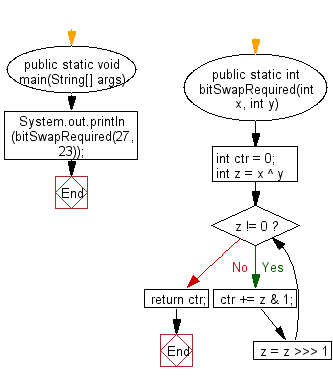﻿ Java: Number of bits required to flip to convert 2 integers# Java Exercises: Find the number of bits required to flip to convert two given integers

## Java Basic: Exercise-147 with Solution

Write a Java program to find the number of bits required to flip to convert two given integers.

Example: 27 --> 11011
23--> 10111

Sample Solution:

Java Code:

``````public class Solution {
public static void main(String[] args) {
System.out.println(bitSwapRequired(27, 23));
}

public static int bitSwapRequired(int x, int y) {
int ctr = 0;
for (int z = x ^ y; z != 0; z = z >>> 1) {
ctr += z & 1;
}
return ctr;
}
}
```
```

Sample Output:

```2
```

Flowchart:Java Code Editor:

What is the difficulty level of this exercise?

Test your Programming skills with w3resource's quiz.

﻿

## Java: Tips of the Day

getEnumMap

Converts to enum to Map where key is the name and value is Enum itself.

```public static <E extends Enum<E>> Map<String, E> getEnumMap(final Class<E> enumClass) {
return Arrays.stream(enumClass.getEnumConstants())
.collect(Collectors.toMap(Enum::name, Function.identity()));
}
```

Ref: https://bit.ly/3xXcFZt